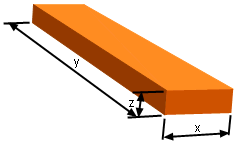Ch 2. Derivatives I Multimedia Engineering Math DerivativeFormulas TrigonometricFunctions Differentiability ChainRule ImplicitDifferentiation
 Chapter 1. Limits 2. Derivatives I 3. Derivatives II 4. Mean Value 5. Curve Sketching 6. Integrals 7. Inverse Functions 8. Integration Tech. 9. Integrate App. 10. Parametric Eqs. 11. Polar Coord. 12. Series Appendix Basic Math Units Search eBooks Dynamics Fluids Math Mechanics Statics Thermodynamics Author(s): Hengzhong Wen Chean Chin Ngo Meirong Huang Kurt Gramoll ©Kurt GramollMATHEMATICS - CASE STUDY IntroductionRectangular Box In his arts and crafts class, Tony is given a piece of paper and asked to make a rectangular box. The length of the box he created is 3 times of its width. His friends made boxes with various lengths, widths and heights. He wonders how the height changes affects the width. What is known: The surface area of the box is constant at 100 in2. The length is 3 times of its width. Questions What is the rate of change of the width with respect to the enlarge of height? Approach Find the relationship between the width and the height. Apply implicit differentiation method